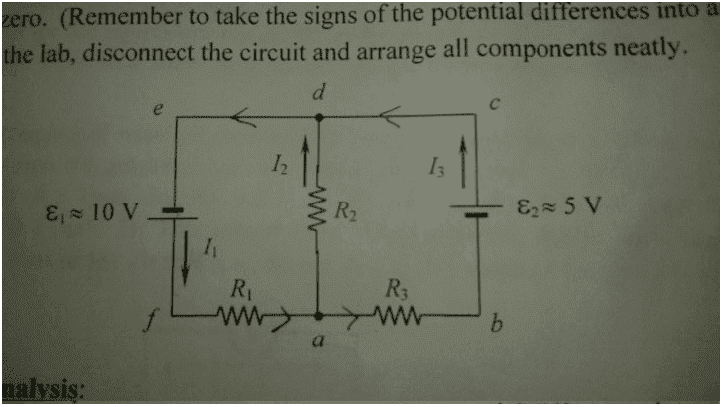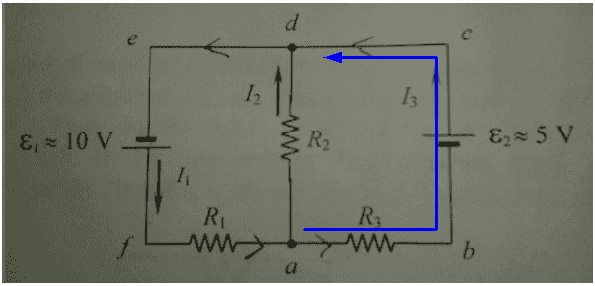# Find currents and voltages - 2 batteries & 3 resistors

kamhogo

## Homework StatementFind I1, I2, I3 as well as the voltages across each resistor.

R1= 50 ohms
R2= 220 ohms
R3= 150 ohms

## Homework Equations

Kirchhoff's loop law
Kirchhoff's junction law

## The Attempt at a Solution

Loop 1: Starting from the negative terminal of the 10V battery (ccw)

10V - 50 (I1) - 220 (I2) = 0

Loop 2: Starting from junction a (ccw). Note I added an I4 through R3 to try and make things easier for me to visualize .

- 150 (I4) + 5 V + 0 (I3) + 220 (I2) = 0

Junction law
I1 = I2 + I4

Loop 1
10V - 50 I2 - 50 I4 - 220 I2 =0
10V - 270 I2 - 50 I4 = 0

Loop 2
I2= (150 I4 - 5 V )/220 = 0.682 I4 - 0.0227 A

Loop 1
10V - 270 (0.682 I4 - 0.0227 A) - 50 I4 = 0
- 234.14 I4 = -16. 129
I4 = 0.069 A
I2= 0.024 A
I1= 0.093 A
I3 = ???????

Voltages across :
R1 ==> 4.65 V
R2 ==> 5.28 V
R3 ==> 10.35 V

I can't find I3. Is it possible that R2 "receives" a portion of I3 downstream (.....I5. ....) in addition to having I2 go through R2 it upstream? ???? Or maybe I3 is infinitely big (ideal wire model, goes from the positive terminal of the 5V battery and then directly to the negative terminal of the 10V battery)???

## Answers and Replies

Mentor
I don't know why you've introduced I4. The branch has two components in series, so they MUST carry the same current, and that's already labelled I3.kamhogo
I don't know why you've introduced I4. The branch has two components in series, so they MUST carry the same current, and that's already labelled I3.

Oooh. I see it now. Thanks!

View attachment 99436

kamhogo
Resolved.

Last edited: